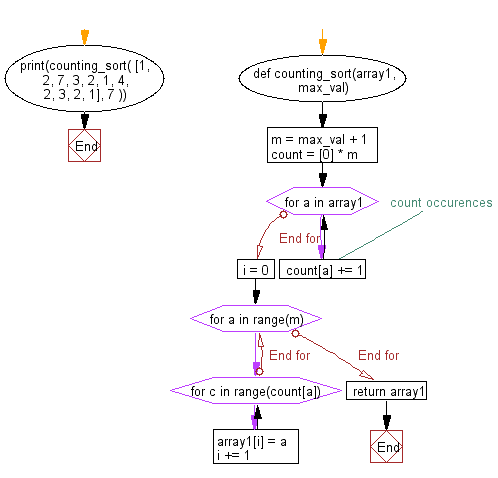﻿ Python: Counting sort algorithm - w3resource# Python: Counting sort algorithm

## Python Search and Sorting: Exercise-10 with Solution

Write a Python program for counting sort.
According to Wikipedia "In computer science, counting sort is an algorithm for sorting a collection of objects according to keys that are small integers; that is, it is an integer sorting algorithm. It operates by counting the number of objects that have each distinct key value, and using arithmetic on those counts to determine the positions of each key value in the output sequence. Its running time is linear in the number of items and the difference between the maximum and minimum key values, so it is only suitable for direct use in situations where the variation in keys is not significantly greater than the number of items. However, it is often used as a subroutine in another sorting algorithm, radix sort, that can handle larger keys more efficiently".

Sample Solution:

Python Code:

``````def counting_sort(array1, max_val):
m = max_val + 1
count =  * m

for a in array1:
# count occurences
count[a] += 1
i = 0
for a in range(m):
for c in range(count[a]):
array1[i] = a
i += 1
return array1

print(counting_sort( [1, 2, 7, 3, 2, 1, 4, 2, 3, 2, 1], 7 ))
```
```

Sample Output:

```[1, 1, 1, 2, 2, 2, 2, 3, 3, 4, 7]
```

Flowchart:## Visualize Python code execution:

The following tool visualize what the computer is doing step-by-step as it executes the said program:

Python Code Editor:

What is the difficulty level of this exercise?

Test your Programming skills with w3resource's quiz.

﻿

## Python: Tips of the Day

Use Reversed() In for Loops:

```>>> tasks = ['laundry', 'picking up kids', 'gardening', 'cooking']